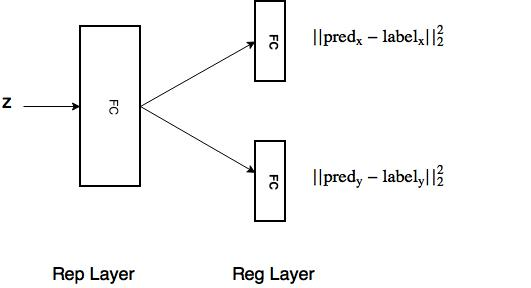# Training a multiple output network

I am trying to build a network similar to this figure:Here is the code for training:

``````import torch
import torchvision
import torch.nn as nn
import numpy as np
import torch.utils.data as data
import torchvision.transforms as transforms
import torchvision.datasets as dsets
import pdb

class RegNet(nn.Module):
def __init__(self):
super(RegNet,self).__init__()
self.Reg_x = nn.Linear(4,4)
self.Reg_y = nn.Linear(4,3)

def forward(self,x):
x_x = self.Reg_x(x)
x_y = self.Reg_y(x)
return x_x,x_y

# Input

# Rep Layer
Rep = nn.Linear(4,4)

# Reg Layer
Reg = RegNet()

label_x = Variable(torch.ones(5,4))
label_y = Variable(torch.zeros(5,3))

criterion = nn.MSELoss()
optimizer1 = torch.optim.SGD(Rep.parameters(), lr=0.01)
optimizer2 = torch.optim.SGD(Reg.parameters(), lr=0.01)

# train
# feed-forward
rep_out = Rep(z)
pred_x,pred_y = Reg(rep_out)

loss_x = criterion(pred_x,label_x)
loss_y = criterion(pred_y,label_y)
loss = loss_x + loss_y

loss.backward()
``````

I have a similar implementation in Torch. On comparing the performance, the predictions pred_y are consistent with Torch implementation while pred_x is way off. Is there something not correct in my implementation.